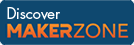Cody

# Problem 12. Fibonacci sequence

Created by Cody Team in Community

Calculate the nth Fibonacci number.

Given n, return f where f = fib(n) and f(1) = 1, f(2) = 1, f(3) = 2, ...

Examples:

``` Input  n = 5
Output f is 5```
``` Input  n = 7
Output f is 13```

### Solution Stats

38.19% Correct | 61.81% Incorrect
Last solution submitted on Mar 25, 2019

#### TagsMATLAB and Simulink resources for Arduino, LEGO, and Raspberry Pi